Definitions of Square Dance Calls and Concepts
Mirror Concept [C3B]

Index -->  Plus  |  A1  |  A2  |  C1  |  C2  |  C3A  |  C3B  |  C4  |  NOL  |
Definitions (Text Only) -->  Plus  |  A1  |  A2  |  C1  |  C2  |  C3A  |  C3B  |  C4  |  NOL  |
 Find call:

 \$B8@8l(BMirror CONCEPT \$B\$O(B, \$BM?\$(\$i\$l\$?%3!<%k\$NDj5A\$r

• Right \$B\$r(B Left \$B\$K(B, \$B\$^\$?5U\$K(B Left \$B\$r(B Right \$B\$K(B, \$B\$=\$7\$F(B,
• Beau \$B\$r(B Belle \$B\$K(B, \$B\$^\$?5U\$K(B Belle \$B\$r(B Beau \$B\$K\$7\$^\$9(B.

 Mirror Swing Thru: Left Swing Thru Mirror Flutter Wheel: Reverse Flutter Wheel Mirror Here Comes The Judge: Sock It To Me Mirror Tag The Line: \$B1&8*\$NBe\$o\$j\$K:87?\$GDL\$j2a\$.\$^\$9(B. Mirror Slide Thru: Left Pass Thru \$B\$r\$7(B; Boys 1/4 Left, Girls 1/4 Right Mirror Wheel & Deal: (One-Faced Line \$B\$+\$i(B): \$B:8B&\$N%+%C%W%k\$,A0\$K\$J\$j\$^\$9(B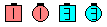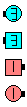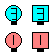Mirror Wheel & Deal\$B\$NA0(B \$BH>J,\$N\$H\$3\$m(B \$B8e(B

Mirror \$B\$G%3!<%k\$r9T\$\$(B, 2 \$B?M\$,F1\$8>l=j\$G=*\$o\$k\$H\$-(B (\$BIaDL\$G\$J\$\$(B Tally-Ho \$B\$N\$h\$&\$J\$H\$-(B), \$B1&Mirror CONCEPT \$B\$OF1\$8>l=j\$G1&

\$BCmLeft \$B\$H(B Reverse \$B\$,(B Mirror \$B\$r<(\$98@MU\$H\$7\$F;H\$o\$l\$F\$-\$^\$7\$?(B (\$BNc\$(\$P(B, Left Swing Thru, Reverse Flutter Wheel), \$B\$7\$+\$7\$=\$l\$i\$N;H\$\$J}\$K\$O0l4S@-\$,\$"\$j\$^\$;\$s(B. 'Left Circle By' \$B\$N\$h\$&\$J%3!<%k\$,\$J\$5\$l\$?\$H\$-(B, \$B%@%s%5!<\$O2?\$r0U?^\$7\$F\$\$\$k\$N\$+J,\$+\$i\$J\$\$\$G\$7\$g\$&(B. Circle Left \$B\$r;O\$a\$N(B fraction \$B\$@\$19T\$\$(B, Left Touch \$B\$rReverse \$B\$O(B 'In Reverse Order', 'Rewind' \$B\$r0UL#\$7(B, \$B\$^\$?B>\$N0UL#\$N\$3\$H(B ('Reverse Stack The Line': \$B%*%j%8%J%k\$N(B Leaders \$B\$G\$J\$/(B Trailers \$B\$,9T\$-(B\$B1&\$B\$r\$H\$j\$^\$9(B!) \$B\$b\$"\$j\$^\$9(B. \$B\$b\$7(B Left \$B\$+(B Reverse \$B\$N\$I\$A\$i\$+\$r;H\$o\$J\$1\$l\$P\$J\$i\$J\$\$\$H\$7\$?\$i(B, Shake & Rattle \$B\$N(B Mirror \$B\$O\$I\$A\$i\$r;H\$&\$G\$7\$g\$&\$+(B? Shakedown \$B\$N(B Mirror \$B\$O(B Left Shakedown \$B\$H%3!<%k\$5\$l(B, Reverse Split Swap \$B\$N(B Mirror \$B\$O(B Split Swap \$B\$H%3!<%k\$5\$l\$^\$9(B! Mirror CONCEPT \$B\$O\$3\$l\$i\$N[#Kf\$5\$r2r7h\$7\$^\$9(B.

\$B%R%s%H(B: Mirror (\$B6@(B) \$B\$,\$"\$kIt20\$NCf\$K\$\$\$FIaDL\$K%3!<%k\$rF0\$\$\$FMY\$C\$F\$\$\$k\$H\$-(B, \$B6@\$NCf\$G\$O%3!<%k\$r(B Mirror \$B\$GMY\$C\$F\$\$\$^\$9(B.

\$B6&DL\$N4V0c\$\$(B: Mirror Pass The Ocean \$B\$O(B Pass The Sea \$B\$G\$O\$"\$j\$^\$;\$s(B. Mirror Pass The Ocean \$B\$G\$O:88*\$N(B Pass Thru \$B\$G\$9\$,(B, Pass The Sea \$B\$G\$O1&8*\$N(B Pass Thru \$B\$G\$9(B.

\$BCm

Mirror Circle By fraction By fraction | anything [C3B]: \$B8~\$\$9g\$C\$?%+%C%W%k\$+\$i(B. Circle Right \$B\$r;O\$a\$N(B fraction \$B\$@\$19T\$\$(B Step To A L-H Wave \$B\$r\$7(B; Arm Turn \$B\$rfraction \$B\$@\$1\$9\$k\$+\$^\$?\$O(B anything \$B\$N%3!<%k\$r9T\$\$\$^\$9(B. \$B\$3\$N%3!<%k\$N(B fraction \$B\$N%G%U%)%k%H\$O\$"\$j\$^\$;\$s(B. \$BNr;KE*\$K\$O(B Reverse Circle By \$B\$H\$7\$FCN\$i\$l\$F\$\$\$^\$9(B.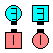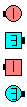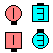Mirror Circle By 1/4 By 1/4\$B\$NA0(B Circle Right 1/4 \$B\$H(B Step To A L-H Wave\$B\$N8e(B Arm Turn 1/4\$B\$N8e(B (\$B=*\$o\$j(B)

Mirror Recycle [C3B]: \$B8~\$\$9g\$C\$?%+%C%W%k\$+\$i(B. Belles \$B\$O(B Extend \$B\$r\$7:82s\$j\$K(B U-Turn Back \$B\$r\$7(B, Beaus \$B\$O(B Belle \$B\$,%3!<%k\$r;O\$a\$k0LCV\$X(B Dodge \$B\$7(B Veer Right \$B\$r\$7\$^\$9(B. \$B:8Reverse Recycle \$B\$H\$7\$FCN\$i\$l\$F\$\$\$^\$9(B.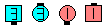Mirror Recycle\$B\$NA0(B \$B8e(B

Mystic CONCEPT [C4]: Center 4 \$B\$O(B Mirror \$B\$G(B Outer 4 \$B\$OIaDL\$K(B, \$BM?\$(\$i\$l\$?%3!<%k\$r9T\$\$\$^\$9(B. \$B\$b\$7(B, Centers \$B\$,\$\$\$D\$+%*%j%8%J%k\$N(B Outsides \$B\$HF0\$/\$3\$H\$,\$"\$k%3!<%k\$N\$H\$-\$O(B, \$B\$=\$l0J9_\$OIaDL\$NJ}K!\$G9T\$\$\$^\$9(B (\$B\$9\$J\$o\$A(B, \$B%*%j%8%J%k\$N(B Centers \$B\$O\$=\$l0J>e(B Mirror \$B\$GF0\$-\$^\$;\$s(B). \$B\$3\$N>l9g(B, \$B%3!<%i!<\$O(B Initially Mystic \$B\$H8@\$C\$?J}\$,NI\$\$\$G\$7\$g\$&(B.Choreography for Mirror ConceptComments? Questions? Suggestions?

https://www.ceder.net/def/mirror.php?level=C3B&language=japan
22-November-2019 12:30:11